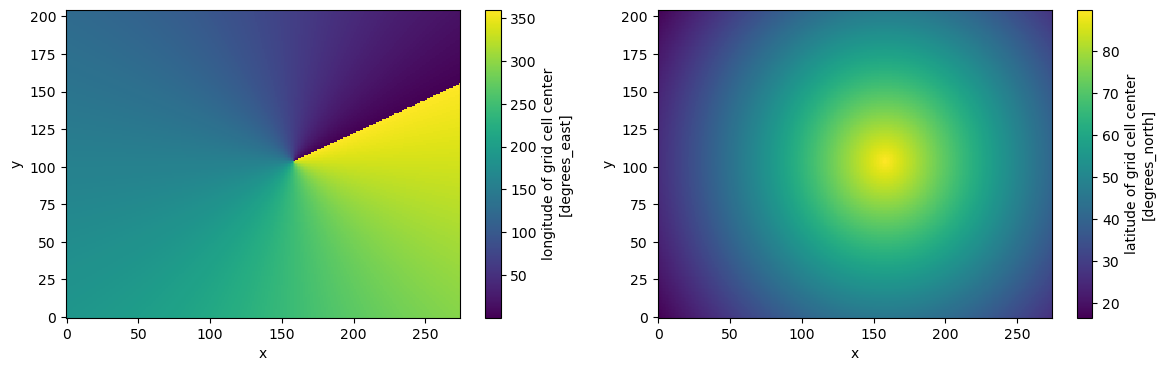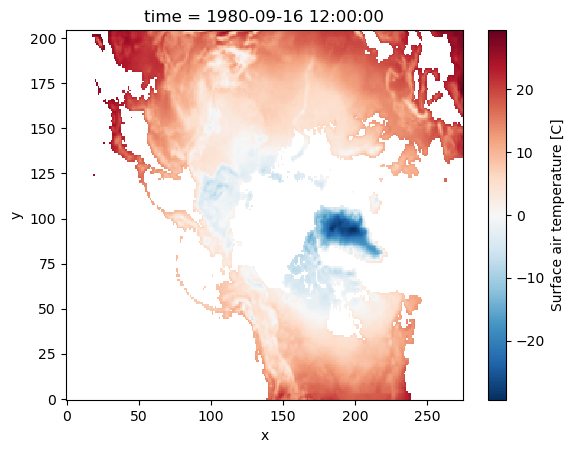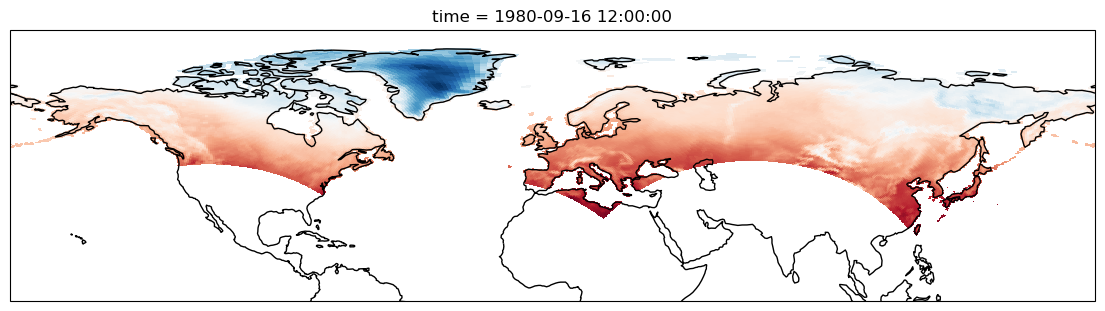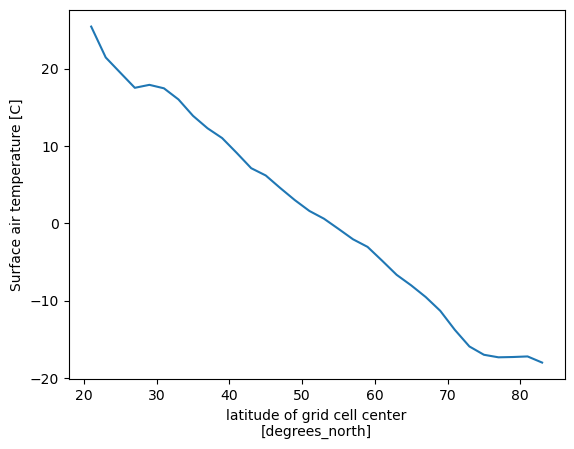You can run this notebook in a live sessionor view it on Github.

# Working with Multidimensional Coordinates#

Author: Ryan Abernathey

Many datasets have physical coordinates which differ from their logical coordinates. Xarray provides several ways to plot and analyze such datasets.

:

%matplotlib inline
import numpy as np
import pandas as pd
import xarray as xr
import cartopy.crs as ccrs
from matplotlib import pyplot as plt


As an example, consider this dataset from the xarray-data repository.

:

ds = xr.tutorial.open_dataset("rasm").load()
ds

:

<xarray.Dataset>
Dimensions:  (time: 36, y: 205, x: 275)
Coordinates:
* time     (time) object 1980-09-16 12:00:00 ... 1983-08-17 00:00:00
xc       (y, x) float64 189.2 189.4 189.6 189.7 ... 17.65 17.4 17.15 16.91
yc       (y, x) float64 16.53 16.78 17.02 17.27 ... 28.26 28.01 27.76 27.51
Dimensions without coordinates: y, x
Data variables:
Tair     (time, y, x) float64 nan nan nan nan nan ... 29.8 28.66 28.19 28.21
Attributes:
title:                     /workspace/jhamman/processed/R1002RBRxaaa01a/l...
institution:               U.W.
source:                    RACM R1002RBRxaaa01a
output_frequency:          daily
output_mode:               averaged
convention:                CF-1.4
references:                Based on the initial model of Liang et al., 19...
comment:                   Output from the Variable Infiltration Capacity...
NCO:                       netCDF Operators version 4.7.9 (Homepage = htt...
history:                   Fri Aug  7 17:57:38 2020: ncatted -a bounds,,d...

In this example, the logical coordinates are x and y, while the physical coordinates are xc and yc, which represent the longitudes and latitudes of the data.

:

print(ds.xc.attrs)
print(ds.yc.attrs)

{'long_name': 'longitude of grid cell center', 'units': 'degrees_east'}
{'long_name': 'latitude of grid cell center', 'units': 'degrees_north'}


## Plotting#

Let’s examine these coordinate variables by plotting them.

:

fig, (ax1, ax2) = plt.subplots(ncols=2, figsize=(14, 4))
ds.xc.plot(ax=ax1)
ds.yc.plot(ax=ax2)

:

<matplotlib.collections.QuadMesh at 0x7f409561e9e0>Note that the variables xc (longitude) and yc (latitude) are two-dimensional scalar fields.

If we try to plot the data variable Tair, by default we get the logical coordinates.

:

ds.Tair.plot()

:

<matplotlib.collections.QuadMesh at 0x7f40953da7a0>In order to visualize the data on a conventional latitude-longitude grid, we can take advantage of xarray’s ability to apply cartopy map projections.

:

plt.figure(figsize=(14, 6))
ax = plt.axes(projection=ccrs.PlateCarree())
ax.set_global()
ds.Tair.plot.pcolormesh(
)
ax.coastlines()
ax.set_ylim([0, 90]);## Multidimensional Groupby#

The above example allowed us to visualize the data on a regular latitude-longitude grid. But what if we want to do a calculation that involves grouping over one of these physical coordinates (rather than the logical coordinates), for example, calculating the mean temperature at each latitude. This can be achieved using xarray’s groupby function, which accepts multidimensional variables. By default, groupby will use every unique value in the variable, which is probably not what we want. Instead, we can use the groupby_bins function to specify the output coordinates of the group.

:

# define two-degree wide latitude bins
lat_bins = np.arange(0, 91, 2)
# define a label for each bin corresponding to the central latitude
lat_center = np.arange(1, 90, 2)
# group according to those bins and take the mean
Tair_lat_mean = ds.Tair.groupby_bins("yc", lat_bins, labels=lat_center).mean(
dim=xr.ALL_DIMS
)
# plot the result
Tair_lat_mean.plot()

:

[<matplotlib.lines.Line2D at 0x7f4094210ca0>]The resulting coordinate for the groupby_bins operation got the _bins suffix appended: yc_bins. This help us distinguish it from the original multidimensional variable yc.

Note: This group-by-latitude approach does not take into account the finite-size geometry of grid cells. It simply bins each value according to the coordinates at the cell center. Xarray has no understanding of grid cells and their geometry. More precise geographic regridding for xarray data is available via the xesmf package.

[ ]: